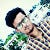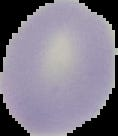# Image analysis for Malaria detection using Convolutional Neural Network

Source: Deep Learning on Medium

### Abstract

Malaria is a life-threatening disease caused by parasites that are transmitted to people through the bites of infected mosquitoes. Automation of the diagnosis process will enable accurate diagnosis of the disease and hence holds the promise of delivering reliable healthcare to resource-scarce areas. Machine learning technologies have been used for automated diagnosis of malaria. We present some of our recent progresses on highly accurate classification of malaria-infected cells using deep convolutional neural networks. First, we describe image processing methods used for segmentation of red blood cells from wholeslide images. We then discuss the procedures of compiling a pathologists-curated image dataset for training deep neural network, as well as data augmentation methods used to significantly increase the size of the dataset, in light of the overfitting problem associated with training deep convolutional neural networks. We will then compare the classification accuracies obtained by deep convolutional neural networks through training, validating, and testing with various combinations of the datasets. These datasets include the original dataset and the significantly augmented datasets, which are obtained using direct interpolation, as well as indirect interpolation using automatically extracted features provided by stacked autoencoders. This chapter ends with a discussion of further research.

### Keywords:

deep learning, convolutional neural network, autoencoders, data augmentation, classification, wholeslide images, malariaParasitized Cell & Uninfected Cell

#### Cell image pre-processing and compilation of dataset for deep learning

The images used in this work were whole slide images provided in the PEIR-VM repository built by the University of Alabama in Birmingham. The original whole slide image data contain significant amount of redundant information. In order to achieve good classification accuracy, image segmentation and de-noising are needed to extract only blood cells and remove those redundant image pixels simultaneously. Several effective image processing techniques were used to accurately segment tiles into individual cells.

#### Image pre-processing tasks

Most image tiles may easily be visualized as having no malaria-infected cells, so preselection of noninfected tiles can be used to significantly reduce overall processing runtime. Given the contrast between the darkly purple/blue-stained nuclei of malaria and the light pink color of normal cells, pixel color information is used for preliminary selection of “infected” tiles. In order to estimate the color of infected cells, we conducted statistical analysis on the collected cell pixels. The maximal and minimal RGB values of infected cells were selected as two thresholds for “suspect” tiles. Considering the risk of excluding infected cells, we expanded the selected RGB value range to include more tiles. In this work, 24,648 of the original 85,094 tiles (29%) were marked as suspect and require further analysis. For the suspected tile, thresholding is performed on the binarized image using Otsu’s method. We can see that noise not only exists in the image background but also inside RBCs. A series of morphological steps were applied to fill the isolated dots and holes to finally obtain the individual cell samples. In our work, only RBCs will provide features in the wholeslide image to the following classification. Therefore, we only keep RBCs and remove everything else using a combination of morphological operations. After all RBCs are processed, we then obtain all clean RBC samples for further classification. Figure 3 shows some normal and infected RBC samples.

### Keras Implentation

#### Keras libraries

from keras.models import Sequential
from keras.layers import Conv2D
from keras.layers import MaxPooling2D
from keras.layers import Flatten
from keras.layers import Dense

#### Part 1 — Initialising the CNN

classifier = Sequential()

#Step 1 — Convolution
classifier.add(Conv2D(32, (3, 3), input_shape = (64, 64, 3), activation = ‘relu’))

# Step 2 — Pooling
classifier.add(MaxPooling2D(pool_size = (2, 2)))

# Adding a second convolutional layer
classifier.add(Conv2D(32, (3, 3), activation = ‘relu’))
classifier.add(MaxPooling2D(pool_size = (2, 2)))
#adding 3rd layer
classifier.add(Conv2D(32, (3, 3), activation = ‘relu’))
classifier.add(MaxPooling2D(pool_size = (2, 2)))

# Step 3 — Flattening
classifier.add(Flatten())

# Step 4 — Full connection
classifier.add(Dense(units = 128, activation = ‘relu’))
classifier.add(Dense(units = 1, activation = ‘sigmoid’))

# Compiling the CNN
classifier.compile(optimizer = ‘adam’, loss = ‘binary_crossentropy’, metrics = [‘accuracy’])

#### Part 2 — Fitting the CNN to the images

from keras.preprocessing.image import ImageDataGenerator

train_datagen = ImageDataGenerator(rescale = 1./255,
shear_range = 0.2,
zoom_range = 0.2,
horizontal_flip = True)

test_datagen = ImageDataGenerator(rescale = 1./255)

training_set = train_datagen.flow_from_directory(‘cell_images/train’,
target_size = (64, 64),
batch_size = 32,
class_mode = ‘binary’)

test_set = test_datagen.flow_from_directory(‘cell_images/test’,
target_size = (64, 64),
batch_size = 32,
class_mode = ‘binary’)

classifier.fit_generator(training_set,
steps_per_epoch = 5000,
epochs = 10,
validation_data = test_set,
validation_steps = 2000)

#### Accuracy :

Validation Accuracy 97%

#### Reference

Keras Documentation — https://keras.io

Classification of Malaria-Infected Cells Using Deep Convolutional Neural Networks — W. David Pan, Yuhang Dong and Dongsheng Wu